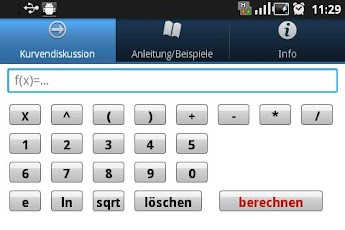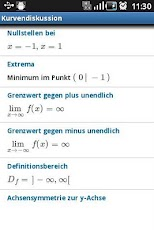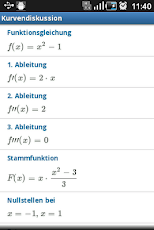# Calculus Curve Sketching## Description

Let your phone do the calculation!

This is the definitive app for calculus!

Simply insert your function into The Calculus Curve Sketching App.

Features:

- derivative
- second derivative
- third derivative
- antiderivatives
- zeros of the function
- singularities
- extrema
- inflections points
- limit as x approaches infinity
- limit as x approaches a specific value
- domain of a function
- asymptotes
- symmetry

Calculation methods are not displayed, only results for comparing your calculations.

List of supported types of functions:

- Polynomial functions
- Rational functions
- Root functions
- Logarithm functions
- Exponential functions
- Sine, cosine and tangent functions

## ScreenshotsVersion 1.1 Uploader Hoster Blackcrowned mediafire.comDownload